# AP Calculus AB : Understanding the limiting process.

## Example Questions

← Previous 1 3

### Example Question #171 : Functions, Graphs, And Limits

Find the derivative.

y = sec (5x3)

y' = –csc(5x3)cot(5x3)

y' = –sec(5x3)tan(5x3)(15x2)

y' = –csc(5x3)cot(5x3)(15x2)

y' = sec(5x3)tan(5x3)(15x2)

y' = sec(5x3)tan(5x3)

y' = sec(5x3)tan(5x3)(15x2)

Explanation:

The derivative of the function y = sec(x) is sec(x)tan(x). First take the derivative of the outside of the function: y = sec(4x3) : y' = sec(5x3)tan(5x3). Then take the derivative of the inside of the function: 5x3 becomes 15x2. So your final answer is: y' = ec(5x3)tan(5x3)15x2

### Example Question #172 : Functions, Graphs, And Limits

Find the slope of the tangent line to the graph of f at x = 9, given that f(x) = –x+ 5√(x)

18

–18

–18 + (5/6)

18 + (5/6)

–18 – (5/6)

–18 + (5/6)

Explanation:

First find the derivative of the function.

f(x) = –x2 + 5√(x)

f'(x) = –2x + 5(1/2)x–1/2

Simplify the problem

f'(x) = –2x + (5/2x1/2)

Plug in 9.

f'(3) = –2(9) + (5/2(9)1/2)

= –18 + 5/(6)

### Example Question #173 : Functions, Graphs, And Limits

Find the derivative

(x + 1)/(x – 1)

1

(–2)/(x + 1)2

(–2)/(x – 1)

(x + 1) + (x – 1)

(–2)/(x – 1)2

(–2)/(x – 1)2

Explanation:

Rewrite problem.

(x + 1)/(x – 1)

Use quotient rule to solve this derivative.

((x – 1)(1) – (x + 1)(1))/(x – 1)2

(x – 1) – x – 1)/(x – 1)2

–2/(x – 1)2

### Example Question #174 : Functions, Graphs, And Limits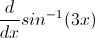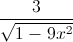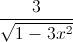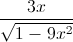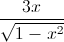Explanation:

Use the chain rule and the formula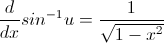### Example Question #1 : Understanding The Limiting Process.

Find the derivative of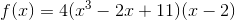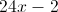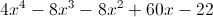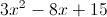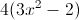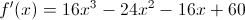Explanation:

The answer is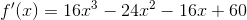. It is easy to solve if we multiply everything together first before taking the derivative.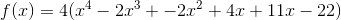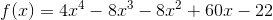### Example Question #176 : Functions, Graphs, And Limits

Differentiate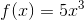.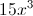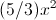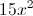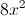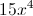Explanation:

Using the power rule, multiply the coefficient by the power and subtract the power by 1.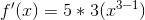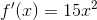### Example Question #177 : Functions, Graphs, And Limits

Differentiate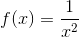.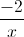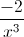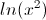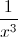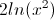Explanation:

Use the product rule: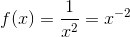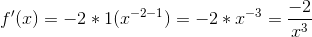### Example Question #1 : Understanding The Limiting Process.

Differentiate: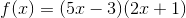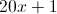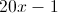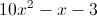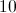Explanation:

Use the product rule to find the derivative of the function.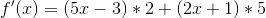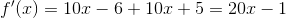### Example Question #2 : Understanding The Limiting Process.

Differentiate: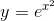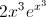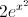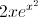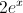Explanation:

The derivative of any function of e to any exponent is equal to the function multiplied by the derivative of the exponent.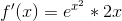### Example Question #3 : Understanding The Limiting Process.

Find the second derivative of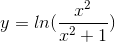.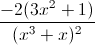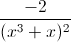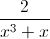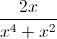Explanation: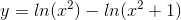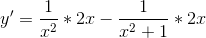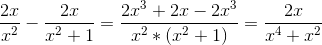Factoring out an x gives you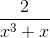.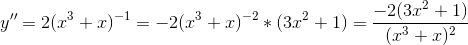← Previous 1 3